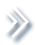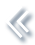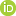####ArchivesReview Articles

## Moderate deviation principle for stochastic reaction-diffusion systems with multiplicative noise and non-Lipschitz reaction

Juan Yang

School of Science, Beijing University of Posts and Telecommunications, Beijing, People's Republic of Chinajuanyang@bupt.edu.cn

Pages | Received 01 Jul. 2020, Accepted 05 Jul. 2021, Published online: 27 Jun. 2022,
• Abstract
• References
• Citations

In this article, we obtain a central limit theorem and prove a moderate deviation principle for stochastic reaction-diffusion systems with multiplicative noise and non-Lipschitz reaction term.

• Budhiraja, A., & Dupuis, P. (2000). A variational representation for positive functionals of infinite dimensional Brownian motion. Probability and Mathematical Statistics-Wroclaw University20(1), 39–61. https://doi.org/10.1080/13550280152537111
• Budhiraja, A., Dupuis, P., & Ganguly, A. (2016). Moderate deviation principle for stochastic differential equations with jump. Annals of Probability44(3), 1–40. https://doi.org/10.1214/15-AOP1007
• Cerrai, S. (2003). Stochastic reaction-diffusion systems with multiplicative noise and non-Lipschitz reaction term. Probability Theory and Related Fields125(2), 271–304. https://doi.org/10.1007/s00440-002-0230-6
• Cerrai, S., & Röckner, M. (2004). Large deviations for stochastic reaction-diffusion systems with multiplicative noise and non-Lipschitz reaction term. Annals of Probability32(1), 1–40. https://doi.org/10.1214/aop/1079021473
• Cerrai, S., & Röckner, M. (2005). Large deviations for invariant measures of stochastic reaction-diffusion systems with multiplicative noise and non-Lipschitz reaction term. Annales de l'Institut Henri Poincare (B) Probability and Statistics41(1), 69–105. https://doi.org/10.1016/j.anihpb.2004.03.001
• Chen, X. (1990). Probabilities of moderate deviations for B-valued independent random vectors. Chinese Journal of Contemporary Mathematics11, 381–393.
• Chen, X. (1991). Probabilities of moderate deviations for independent random vectors in a Banach space. Chinese Journal of Applied Probability and Statistics7, 24–33.
• Chen, X. (1993). On lower bound of the moderate deviations of i.i.d. random variables in Banach space. Chinese Journal of Applied Probability and Statistics9, 386–393.
• Chueshov, I., & Millet, A. (2010). Stochastic 2D hydrodynamics type systems: Well posedness and large deviations. Applied Mathematics and Optimization61(3), 379–420. https://doi.org/10.1007/s00245-009-9091-z
• Cramér, H. (1938). Sur un nouveau théorème limite de la théorie des probabilités. Actualités Scientifiques et Industrielles736, 5–23. https://doi.org/10.1007/978-3-642-40607-2_8
• Davies, E. B. (1989). Heat kernels and spectral theory. Cambridge University Press.
• Dembo, A., & Zeitouni, O. (2000). Large deviations techniques and applications. Springer-Verlag.
• Djellout, H., & Guillin, A. (2001). Moderate deviations for Markov chains with atom stochastic processes and their applications. Stochastic Processes and Their Applications95(2), 203–221. https://doi.org/10.1016/S0304-4149(01)00100-4
• Dong, Z., Xiong, J., Zhai, J. L., & Zhang, T. S. (2017). A moderate deviation principle for 2-D stochastic Navier-Stokes equations driven by multiplicative Lévy noises. Journal of Functional Analysis272(1), 227–254. https://doi.org/10.1016/j.jfa.2016.10.012
• Douc, R., Guillin, A., & Najim, J. (2001). Moderate deviations for particle filtering. The Annals of Applied Probability15(1B), 587–614. https://doi.org/10.1214/105051604000000657
• Gao, F. Q. (1996). Moderate deviations for martingales and mixing random processes. Stochastic Processes and Their Applications61(2), 263–275. https://doi.org/10.1016/0304-4149(95)00078-X
• Gao, F. Q. (2003). Moderate deviations and large deviations for kernel density estimators. Journal of Theoretical Probability16(2), 401–418. https://doi.org/10.1023/A:1023574711733
• Gao, F. Q., & Zhao, X. (2011). Delta method in large deviations and moderate deviations for estimators. The Annals of Statistics39(2), 1211–1240. https://doi.org/10.1214/10-AOS865
• Guillin, A., & Liptser, R. (2006). Examples of moderate deviation principle for diffusion processes. Discrete and Continuous Dynamical Systems -- Series B6(4), 803–828. https://doi.org/10.3934/dcdsb.2006.6.803
• Ledoux, M. (1992). Sur les déviations modérées des sommes de variables aléatoires vectorielles indépendants de même loi. Annales de l'IHP Probabilités et statistiques28, 267–280.
• Wang, R., & Zhang, T. S. (2015). Moderated deviations for stochastic reaction-diffusion equations with multiplicative noise. Potential Analysis42(1), 99–113. https://doi.org/10.1007/s11118-014-9425-6
• Wang, R., Zhai, J. L., & Zhang, T. S. (2015). A moderate deviation principle for 2-D stochastic Navier-Stokes equations. Journal of Differential Equations258(10), 3363–3390. https://doi.org/10.1016/j.jde.2015.01.008
• Wu, L. M. (1995). Moderate deviations of dependent random variables related to CLT. Annals of Probability23(1), 420–445. https://doi.org/10.1214/aop/1176988393
• Wu, L. M. (1999). Forward-Backward martingale decomposition and compactness results for additive functionals of stationary ergodic Markov processes. Annales De L'Institut Henri Poincare (B) Probability and Statistics35(2), 121–141. https://doi.org/10.1016/S0246-0203(99)80008-9

To cite this article: Juan Yang (2022): Moderate deviation principle for stochastic reaction-diffusion
systems with multiplicative noise and non-Lipschitz reaction, Statistical Theory and Related Fields,
DOI: 10.1080/24754269.2021.1963183